# NCERT Solutions for Class 8 Maths Chapter 11 Mensuration InText Questions

These NCERT Solutions for Class 8 Maths Chapter 11 Mensuration InText Questions and Answers are prepared by our highly skilled subject experts.

## NCERT Solutions for Class 8 Maths Chapter 11 Mensuration InText Questions

NCERT Intext Question Page No. 170
Question 1.
(a) Match the following figures with their respective areas in the box.(b) Write the perimeter of each shape.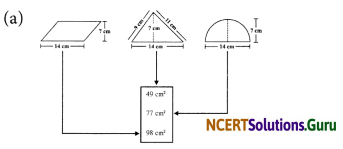(b)
(i)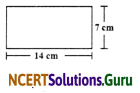The given figure is a rectangle in which
Length = 14cm
∵Perimeter of a rectangle = 2 × [Length + Breadth]
∴ Perimeter of the given figure = 2 × [14cm + 7cm]

(ii)The figure is a square housing its side as 7cm. y
∵ Perimeter of a square = 4 × side
∴ Perimeter of the given figure = 4 × 7cm = 28cmNCERT Intext Question Page No. 172
Question 1.
Nazma’s sister also has a trapezium shaped plot. Divide it into three parts as shown (Fig 11.4). Showthat the area of trapezium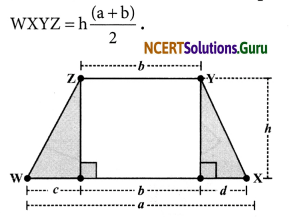Area of ΔPWZ
= $$\frac { 1 }{ 2 }$$ × Base × Altitiude = $$\frac { 1 }{ 2 }$$ × c × h = $$\frac { 1 }{ 2 }$$ch
Area of the rectangle PQYZ = b × h = bh
Area of ΔQXY = $$\frac { 1 }{ 2 }$$ × d × h = $$\frac { 1 }{ 2 }$$dh
∴ Area of the trapezium XYZW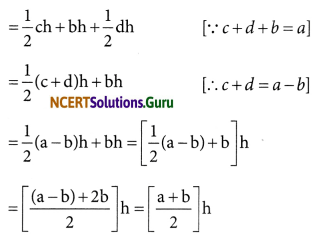Question 2.
If h = 10 cm, c = 6 cm, b = 12 cm, d = 4 cm, find the values of each of its parts separetely and add to find the area WXYZ. Verify it by putting the values of h, a and b in the expression $$\frac{h(a+b)}{2}$$ .
Area of ΔPWZ = $$\frac { 1 }{ 2 }$$ch = $$\frac { 1 }{ 2 }$$ x 6 x 10cm2
= 30cm2
Area of ΔQXY = $$\frac { 1 }{ 2 }$$ dh = $$\frac { 1 }{ 2 }$$ x 4 x 10cm2
= 20 cm2
Area of rectangle PQYZ = Length x Breadth = b x h = 12 x 10cm2 = 120cm2
Area of trapezium WXYZ = Area of ΔPWZ + Area of ΔQXY+Area of rectangle PQYZ
= 30cm2 + 20cm2 + 120cm2 = 170cm2 Also, area of the trapezium WXYZ
= $$\frac{h(a+b)}{2}$$
= $$\frac{10(22+12)}{2}$$ cm2 [a = c + b + d = 6cm + 12cm + 4cm = 22cm]
= $$\frac{10 \times 34}{2}$$ cm2 = 170 cm2
Hence, the area of trapezium is verified.NCERT Intext Question Page No. 172
Question 1.
We know that parallelogram is also a quadrilateral. Let us also split such a quadrilateral into two triangles, find their areas and hence that of the parallelogram. Does this agree with the formula that you know already? (Fig 11.12)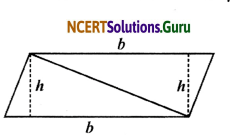The diagonal BD of quadrilateral ABCD is joined and it divides the quadrilateral into two triangles.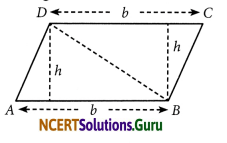Now,
= Area of ΔABD + Area of ΔBCD
= $$\frac { 1 }{ 2 }$$b × h)+ ($$\frac { 1 }{ 2 }$$ b × h) = 2($$\frac { 1 }{ 2 }$$ b × h) = bh
In fact ABCD is a parallelogram.
∴ Area of a parallelogram ABCD = b × h
Area of a parallelogram = Base × Height
We know that a parallelogram can also be a trapezium. We already know that
Area of trapezium ABCD
= $$\frac { 1 }{ 2 }$$ × (Sum of parallel sides) × (Perpendicular distance between parallel sides)
= $$\frac { 1 }{ 2 }$$ × (b + b) × h = $$\frac { 1 }{ 2 }$$ × (2b) × h = bh
or Area of parallelogram ABCD = bh
Yes, the above relation agrees with formula that we know already.

NCERT Intext Question Page No. 175
Question 1.
A parallelogram is divided into two congruent triangles by drawing a diagonal across it. Can we divide a trapezium into two congruent triangles?
No, a trapezium cannot be divided into two congruent triangles.NCERT Intext Question Page No. 176
Question 1.
Divide the following polygons (Fig 11.17) into parts (triangles and trapezium) to find out its area.(a) We draw perpendiculars from opposite vertices on FI, i.e. GL ⊥ FI, HM ⊥ FI and EN⊥ FI
Area of the polygon EFGHI
= ar (ΔGFL) + ar (trapezium GLMH) + ar (ΔHMI) + ar (ΔNEI) + ar (ΔEFN)(b) NQ is a diagonal. Draw OA ⊥ NQ, MB ⊥ NQ, PC ⊥NQ and RD ⊥ NQ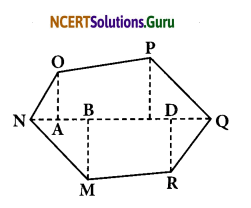∴ Area of Polygon OPQRMN
= ar (ΔOAN) + ar (trap. CPOA) + ar (ΔPCQ) + ar (ΔRDQ) + ar (trap. MBDR) + ar (ΔMBN)Question 2.
Polygon ABCDE is divided into parts as shown below (Fig 11.18). Find its area if AD = 8 cm, AH = 6 cm, AG = 4 cm, AF = 3 cm and perpendiculars BF = 2 cm, CH = 3 cm, EG = 2.5 cm.Area of polygon ABCDE = area of ΔAFB + ….
Area of ΔAFB = $$\frac { 1 }{ 2 }$$ × AF × BF
= $$\frac { 1 }{ 2 }$$ × 3 × 2 = …
Area of trapezium FBCH = FH × $$\frac{(\mathrm{BF}+\mathrm{CH})}{2}$$
= 3 × $$\frac{(2+3)}{2}$$ [FH = AH – AF]
Area of ΔCHD = $$\frac { 1 }{ 2 }$$ × HD × CH = ….
Area of ΔADE = $$\frac { 1 }{ 2 }$$ × AD × GE = ….
So, the area of polygon ABCDE = ….
Area of polygon ABCDE = Area of ΔAFB + Area of ΔADE
Area of ΔAFB = $$\frac { 1 }{ 2 }$$ × AF × BF
= $$\frac { 1 }{ 2 }$$ × 3 × 2 = 3cm2
Area of trapezium FBCH = FH × $$\frac{[\mathrm{BF}+\mathrm{CH}]}{2}$$
= 3 × $$\frac{(2+3)}{2}$$)cm2 [∵ FH = AH – AF]
3 × $$\frac{5}{2}$$ cm2 = $$\frac{15}{2}$$ cm2 = 7.5 cm2
Area of ΔCHD= $$\frac { 1 }{ 2 }$$ × HD × CH
= $$\frac { 1 }{ 2 }$$ × (AD – AH) × CH [∵HD = AD – AH]
= $$\frac { 1 }{ 2 }$$ × (8 – 6) × 3cm2 = 3cm2
Area of ΔADE = $$\frac { 1 }{ 2 }$$ × AD × GE
= $$\frac { 1 }{ 2 }$$ × 8 × 2.5cm2 = 4 × 2.5cm2 = 10cm2
So, the area of polygon ABCD = 3cm2 + 7.5cm2 + 3cm2 + 10cm2 = 23.5cm2

error: Content is protected !!